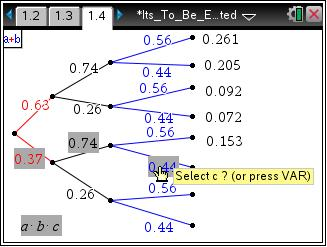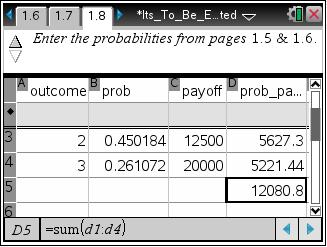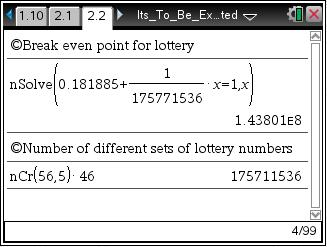### Mathematics lessons for IB® Diploma Programme

Applications and Interpretation | Statistics and Probability## It’s to Be Expected

### Overview

Students use a tree diagram to find theoretical probabilities and use this information in a spreadsheet to find the expected value.

## Key Steps## Step 1

Students will modify a tree diagram to model three basketball players making a shot for a charity contest.## Step 2

Students will be introduced to expected value. The results of the contest are associated with different dollar amounts.## Step 3

The extension involves the application of expected value. Students will use a spreadsheet to calculate probabilities of winning the lottery by matching six numbers. Then they will find the expected value for a given jackpot.